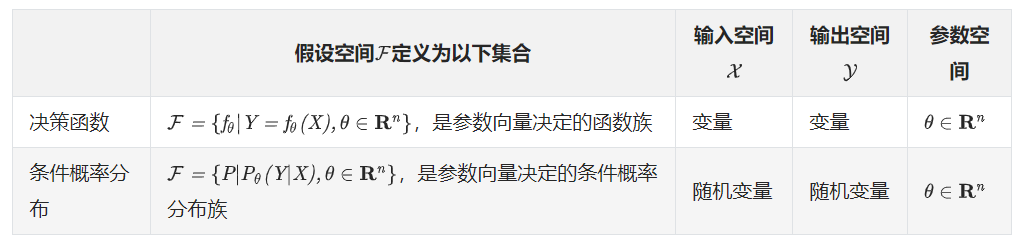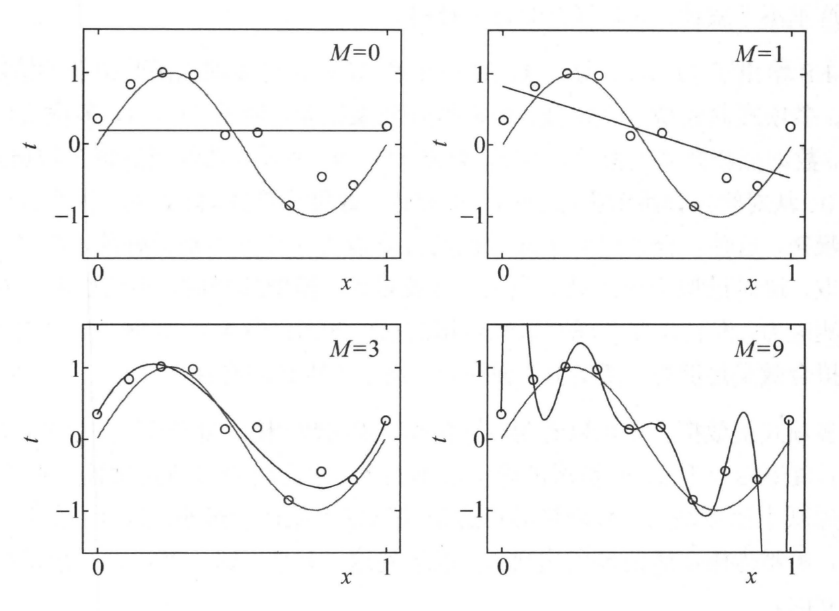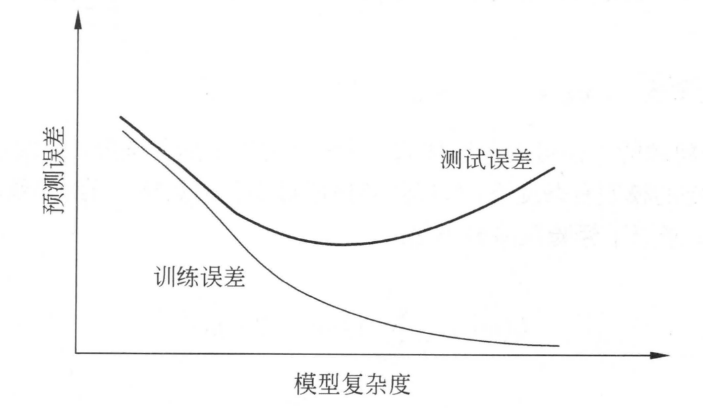# CH01 统计学习及监督学习概论

## 前言

### 导读

• ~~直接看目录结构，会感觉有点乱，就层级结构来讲感觉并不整齐。~~第二版重新梳理了这部分目录结构，舒服多了，尤其之前的分类，回归与标注因为出现了1.10造成目录中这部分不对齐，非常不爽。结果，第二版改了。赞

• 本章最后的三个部分，这三个问题可以对比着看，如果暂时没有概念，略过也可以，回头对各个算法有了感觉回头再看这里。
这三部分怎么对比，三部分都有个图来说明，仔细看下差异，本文后面会对此展开。

• 关于损失函数，风险函数与目标函数注意体会差异

• 后面插点从深度学习角度拿到的点

• 关于机器学习三要素, 复旦大学邱锡鹏教授也有解读[^2]: 模型, 学习准则, 优化算法. 这个定义比较接近代码. 以Tensorflow为例. 通常会定义一个网络(模型), 定义Loss(学习准则), 定义优化算法(Optimizer), 然后开Session, 不停的把数据带入用Opitmizer去最小化Loss.
• Losses, Metrics, 在Keras里面划分了两个模块, 解释是Losses是BP过程用到的, 而Metrics实际和损失函数类似, 用来评价模型的性能, 但是不参与反向传播. 从源码也能看到, Metrics里面import了很多Loss算法
• 书中例子1.1可以参考PRML中对应的表述， 更详细些。

• 在监督学习中输入和输出对称为样本，在无监督学习中输入是样本

• 注意在介绍输入空间，输出空间等概念的时候，以及这一章的很多部分都会有个帽子，监督学习中， 书中也明确了本书主要讨论监督学习的问题，最后的概要总结部分对监督学习有这样的描述：监督学习可以概括如下：从给定有限的训练数据出发，假设数据是独立同分布的，而且假设模型属于某个假设空间，应用某一评价准则，从假设空间中选取一个最优的模型，使它对已给的训练数据以及未知测试数据在给定评价标准意义下有最准确的预测。，理解下这里的假设。

• 在贝叶斯学习部分，提到将模型、为观测要素及其参数用变量表示，使用模型的先验分布是贝叶斯学习的特点。注意这里面先验是模型的先验分布。

• 在泛化误差部分，用了$\hat f$表示最优估计，这个有时候也会用$f^*$表示意思差不多。有时候表示向量又要表示估计值，用$*$可能好看一点，比如$\vec x^*$，但是通常没本书都有自己的符号体系，向量可以通过字体表示，具体可以从书中的符号表部分了解。关于这一点，在第二版第一章就有所体现，监督和无监督学习中，模型用hat表示，在强化学习中，最优解用*表示。

• 提一下参考文献，几个大部头都在，ESL，PRML，DL，PGM，西瓜书，还有Sutton的强化学习，不过这本书2018年出了第二版，感兴趣的话可以看新版。

## 1.1 统计学习

1. 得到一个有限的训练数据集合
2. 确定包含所有可能的模型的假设空间，即学习模型的集合
3. 确定模型选择的准则，即学习的策略
4. 实现求解最优模型的算法，即学习的算法
5. 通过学习方法选择最优的模型
6. 利用学习的最优模型对新数据进行预测或分析

## 1.3 统计学习方法三要素

### 1.3.1 模型### 1.3.2 策略

#### 损失函数与风险函数

• 风险函数(risk function)或期望损失(expected loss)

$R_{exp}(f)=E_p[L(Y, f(X))]=\int_{\mathcal X\times\mathcal Y}L(y,f(x))P(x,y)\, {\rm d}x{\rm d}y$

1. 经验风险(empirical risk)或经验损失(empirical loss)

$R_{emp}(f)=\frac{1}{N}\sum^{N}_{i=1}L(y_i,f(x_i))$

1. 结构风险(structural risk)
$R_{srm}(f)=\frac{1}{N}\sum_{i=1}^{N}L(y_i,f(x_i))+\lambda J(f)$
$J(f)$为模型复杂度, $\lambda \geqslant 0$是系数，用以权衡经验风险和模型复杂度。

#### ERM与SRM

1. **经验风险最小化(ERM)**认为经验风险最小的模型就是最优模型。即求解最优化问题$min_{f \in \cal F}R_{emp}(f)$极大似然估计是经验风险最小化的一个例子。

当模型是条件概率分布，损失函数是对数损失函数时，经验风险最小化等价于极大似然估计。

但样本容量过小时会出现过拟合。

2. 结构风险最小化(SRM)为防止过拟合加入了正则化项。即求解最优化问题$min_{f \in \cal F}R_{srm}(f)$贝叶斯估计中的最大后验概率估计是结构风险最小化的一个例子
当模型是条件概率分布，损失函数是对数损失函数，模型复杂度由模型的先验概率表示时，结构风险最小化等价于最大后验概率估计。

#### 常用损失函数

1. 0-1损失
$L(Y,f(X))=\begin{cases}1, Y \neq f(X) \\0, Y=f(X) \end{cases}$
$L(Y,f(X))=(Y-f(X))^2$
3. 绝对损失
$L(Y,f(X))=|Y-f(X)|$
4. 对数损失（对数似然损失）
这里$P(Y|X)\leqslant 1$，对应的对数是负值，所以对数损失中包含一个负号
$L(Y,P(Y|X))=-\log P(Y|X)$

## 1.4 模型评估与模型选择

### 过拟合与模型选择

M次多项式拟合问题例子：$L(w)=\frac{1}{2}\sum\limits_{i=1}^N(f(x_i,w)-y_i)^2$

$L(w)=\frac{1}{2}\sum\limits_{i=1}^N\left(\sum\limits_{j=0}^Mw_jx_i^j-y_i\right)^2=\frac{1}{2}\sum\limits_{i=1}^N(w\cdot x_i-y_i)^2$

$w$求偏导令其为零，得到一系列方程，求解可以用梯度下降或者矩阵分解。

#### 训练误差与测试误差## 1.5 正则化与交叉验证

### 交叉验证

• 简单交叉验证：将数据随机的切分为两部分：训练集和测试集
• S折(K折, K-Fold)交叉验证[^1]：将数据切分为S个互不相交、大小相同的子集，使用其中的S-1个子集做训练集，剩下的一个做测试集。共有S种选择。
• 留一交叉验证：S折中使S=N

## 1.6 泛化能力

• 现实中采用最多的方法是通过测试误差来评价学习方法的泛化能力
• 统计学习理论试图从理论上对学习方法的泛化能力进行分析
• 泛化误差就是学习到的模型的期望风险
• 学习方法的泛化能力往往是通过研究泛化误差的概率上界进行的, 简称为泛化误差上界(generalization error bound)

### 泛化误差上界

1. 是样本容量的函数，样本容量增加时，泛化上界趋于0；举例子就是若世界上共有猫的图片10万亿张，我的数据集有1亿张和有1万张，训练出的模型的泛化上界是不一样的，我的数据集越多，泛化上界越小，数据集数量越趋近于10万亿，泛化上界越趋近于0。
2. 是假设空间容量的函数，假设空间容量越大，模型就越难学；书上的二分类问题例子有助于理解这句话。

## 1.7 生成模型与判别模型

### 生成方法

generative approach，学习联合概率分布$P(X,Y)$，再求条件概率分布$P(Y|X)$

• 生成模型：$P(Y|X) = \frac{P(X,Y)}{P(X)}$

• 收敛速度快, 当样本容量增加时, 学到的模型可以更快收敛到真实模型

• 当存在隐变量时仍可以用

• 典型的有朴素贝叶斯和隐马模型

### 判别方法

discriminative approach

• 直接学习条件概率$P(Y|X)$或者决策函数$f(X)$
• 直接面对预测, 往往学习准确率更高
• 可以对数据进行各种程度的抽象, 定义特征并使用特征, 可以简化学习问题
• 典型的有k近邻、感知机、逻辑斯蒂回归、最大熵、SVM、提升方法、条件随机场等